The 'Completeness Theorem' with more detail here

*********************************************

The completeness theorem - Stringularity’ & the ‘Overtone-Series’

Elsewhere supports this.

The moment of the so-called ‘Big Bang’ is frequently referred to as ‘singularity’.
The word ‘stringularity’ is used here instead to indicate the unity of the ‘harmonic series’.

Embracing everything from quantum to cosmological scales it is: -

[a] latent before the beginning of space-time &
[b] patently a self-referential measurement in space-time (Hz) of
[c] ratios & rates (where the rule is to double the frequency, halve the wave-length)
[d] producing a pre-determined 'major chord' (force majeure) at the beginning
[e] & always remaining true at all scales as
[f] reflexivity (action and reaction)
[g] relativity (time curvature)
[h] structure, synchronicity & sequence
[i] resonance in locality/universality

‘Force Majeure’ - the ‘factuality’ of the ‘Overtone Series’

With no appeal to any external authority, the rates & ratios underlying all waves & particles, self-referentially & deterministically reveal
what simply exists, the factuality of the ‘overtone-series’, or waves-per-unit-time (Hz) creating the space they occupy, as follows: -

[j] when the wave-length is decreased by 50%, the frequency (Hz) is increased by 200% and a ‘perfect-octave’ emerges (as a Hz doubling) . . .
[k] when the wavelength is decreased by 66.66%, the frequency is increased by 300% and a ‘perfect-octave and a perfect fifth’ emerges (as a Hz trebling) . . .
[l] when the wavelength is decreased by 75%, the frequency is increased by 400% and two perfect octaves emerge (as a Hz quadrupling) . . .
[m
] when the wavelength is decreased by 80%, the frequency is increased by 500% two perfect octaves and a major third emerge (as a Hz quintupling) . . .
[n] (and so on) . . .

Lao Tse, Pythagoras, the rational overtone series and also the neg-entropic emergence of irrational Phi

In the Tao Te Ching (Lao Tse) there are 84 verses (7 * 12). Verse 42 reads, ‘from one comes two, from two comes three & from three come the 10,000 things’.
His contemporary Pythagoras demonstrated this in the overtone series in his ‘string experiment’.

When the overtone series is continued over seven Perfect Octaves (2^7 = 128 Hz) it emerges that this number doesn’t quite commute with
twelve Perfect Fifths (1.5^12 = 129.7643 Hz) i.e. 2^7 ≈ 1.5^12. Known as ‘the Pythagorean Comma’, this has been well-known for millennia.

Commuting these, simply requires ‘Well-Tempering’ (WT) the Perfect Fifths (PF).
This has been well-known since the publication of the Well-Tempered Clavichord (J S Bach 1721).

When 2^7 = 1.49830707686^12, both totals commute at 128 Hz. When the ‘the Pythagorean Comma’
(the Hz differences emerging between Perfect & Well-Tempered 5ths) is shown, a fundamental significance is seen . . .

. . . a Hz sequence, covering twelve Perfect Fifths in the Hz time of seven Perfect Octaves, shows that when the Hz differences between
12 Perfect Fifths & 12 Well Tempered Fifths encoded in the Pythagorean Comma are fed-back on themselves,
a Hz value of ‘Phi’ emerges at exactly the Phi-point between the twelfth & the thirteenth steps
.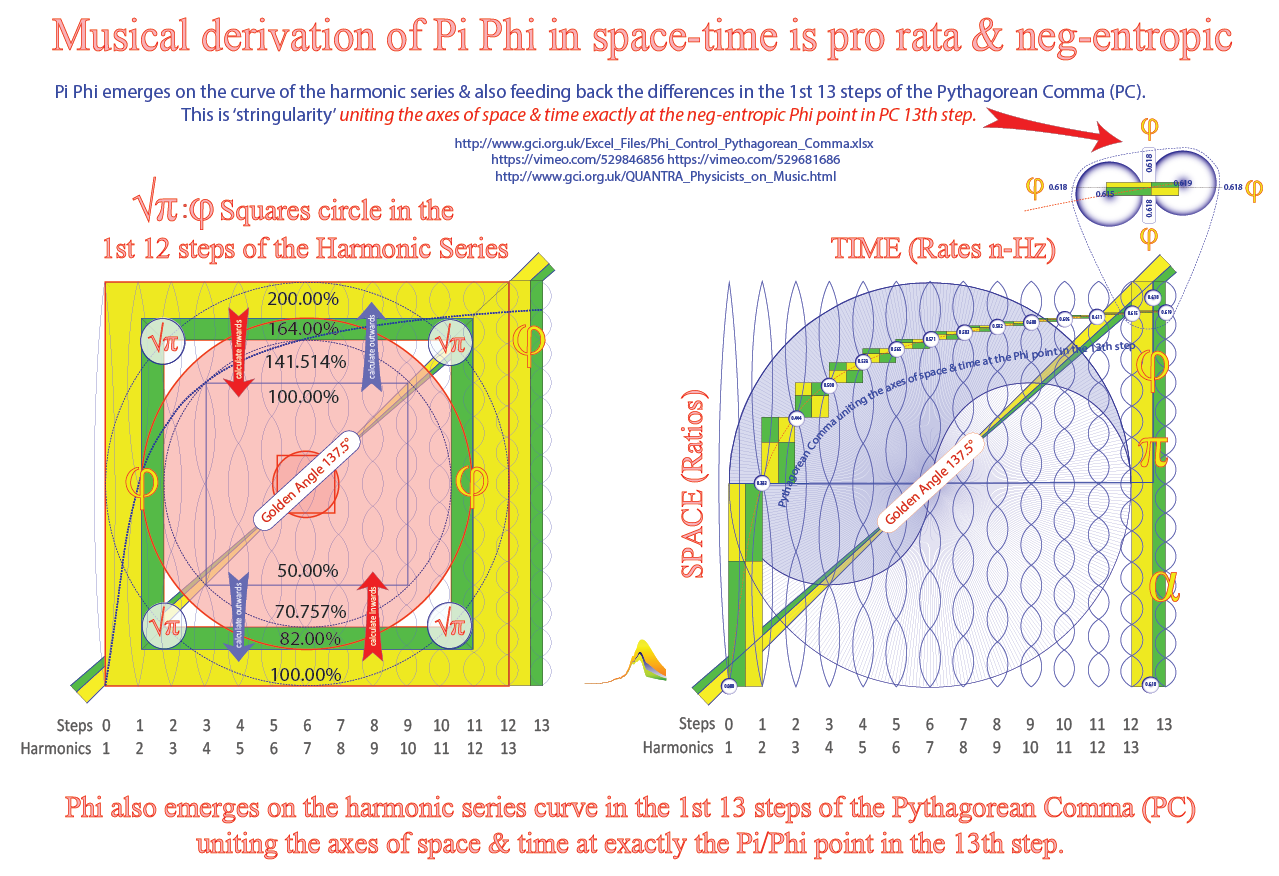This deterministic procedure represents a ‘completeness theorem’ as it is entirely and serendipitously self-referential.
It is an 'Entscheidungsgetroffen'
preceding the ‘Entscheidungsproblem’ or as Gödel might have said, “a kind of miracle”.

The output of this 'factuality' enables the derivatives of diversity.
Supporting detail about the derivatives being developed here

The self-referential nature of this is that it is true at any & at all scales, while the
Phi-derivation remains constant, starting from any control reference value (see model).

Emerging at all scales of space and time, these are correlated and demonstrated elsewhere.
In summary, from this constancy significant issues are signalled, integrating: -

[o] Positive and negative entropy,
[p] Symmetry-breaking and symmetry-binding,
[q] Determinism and in-determinism,
[r] Synchronicity and Sequentiality,
[s]
Universality, locality and entanglement
[t] The context for the values of Pi, Phi & Alpha,
[u] Instability and stability in the Standard Model of Physics,
[v] The double-helix structure of the DNA molecule,
[w] and that from Phyllotaxis, to galaxies and to sub atomic particle decay,
[x] Phi-governed Dynamic Spiral-Structures (DSS) are ubiquitous throughout Nature.

Stringularity underlies the answers to these questions in fundamentals. Integrating the Pi:Phi relationship
in space   (ratios) and in time-space  (rates and ratios) is shown here: -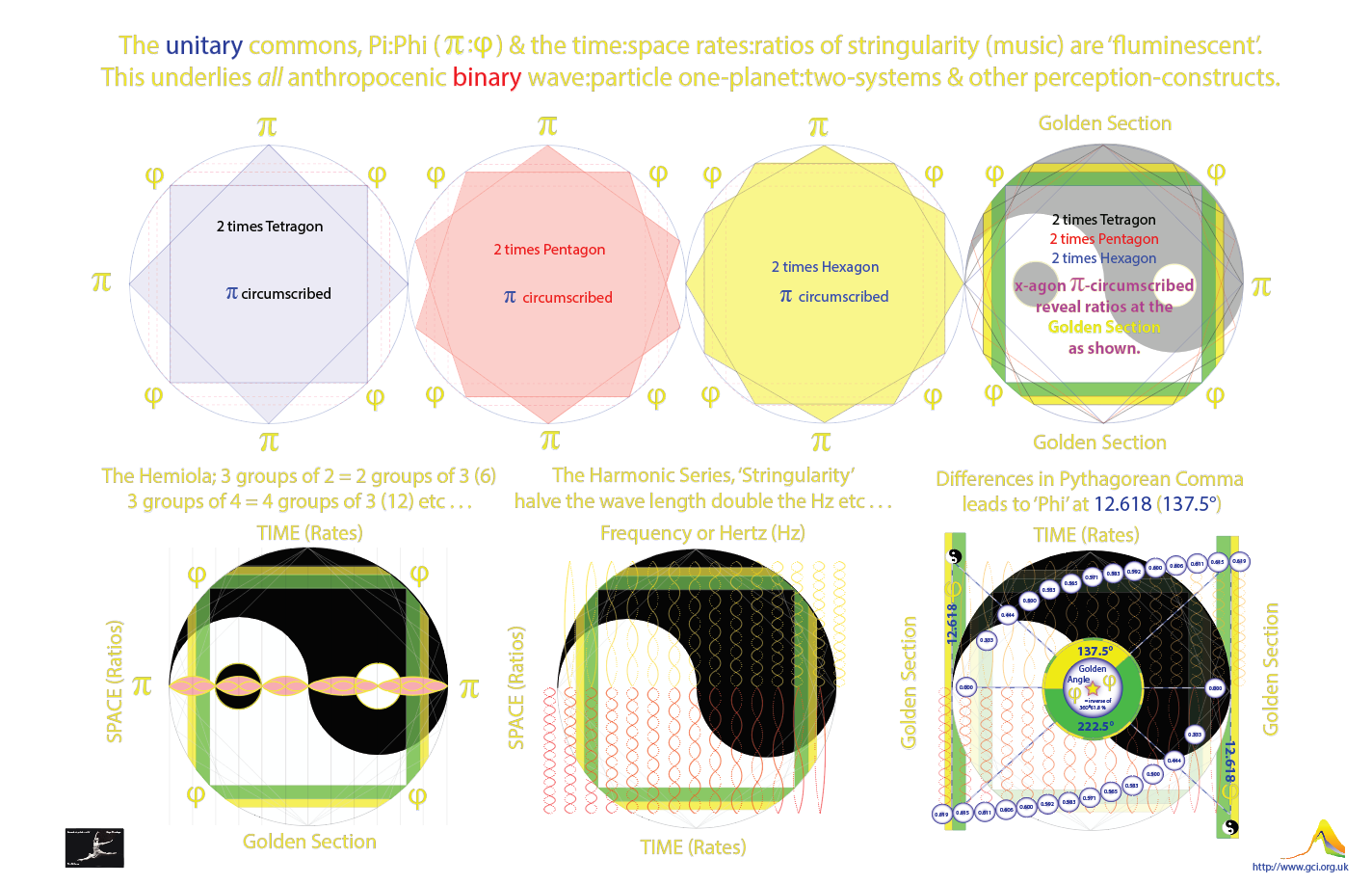As Wilczek says, "Atoms are musical instruments."
Consquently, as musical instruments are governed
by the the overtone series, by definition atoms are governed by the completeness of stringularity.

All musicians and particularly string players (e.g. Albert Einstein) have for eons, innocently and
gratefully depended on stringularity daily in practice and play . . . as nothing exists without it.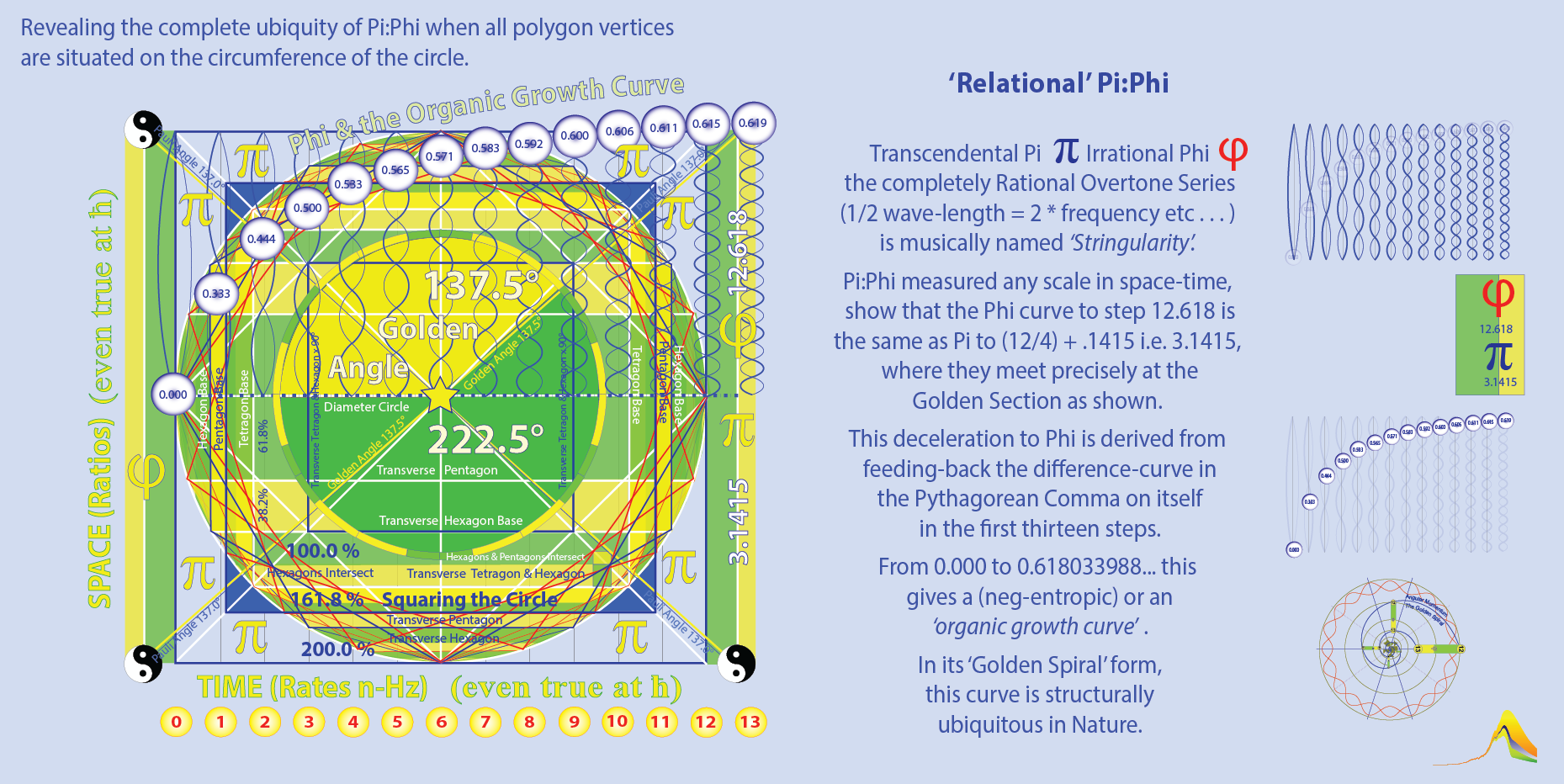Context graphic

***************************************************************

The simple wave form of 'Stringularity' . . . gives rise the 'Trinity' of the 3-2-1 'hemiola',

Length/1 = Hz*1 . . . a Fundamental (here 100 Hz)
Length/2 = Hz*2 . . . a Perfect Octave (a doubling - here 200 Hz) &
Length/3 = Hz*3 . . . a Perfect Octave & a Perfect Fifth (a trebling - here 300 Hz)The hemiola is a simple & stable 'numeraire' . . .

The hemiola is 3 in the time of 2 in the time of 1. It is trinitarian . . . or 2*3 or 3+2+1 = 6/2
The 'frequencies' or pitch rates, coupled with the ratios or proportions of subdivision of these
'bounded-waves' and shorter wave-lengths beyond the hemiola shown are the 'natural-harmonics'.

Symmetry from the 'harmonic-feedback' of the 'hemiola' . . .

For every action there is an equal & opposite reaction (Newton).
Here are advanced & retarded waves, as in Maxwell's equations.

'Forwards & backwards in time', the hemiola is real at all scales of space
time.
The rates:ratios of its wave-symmetry are certain signal & not 'probablistic'.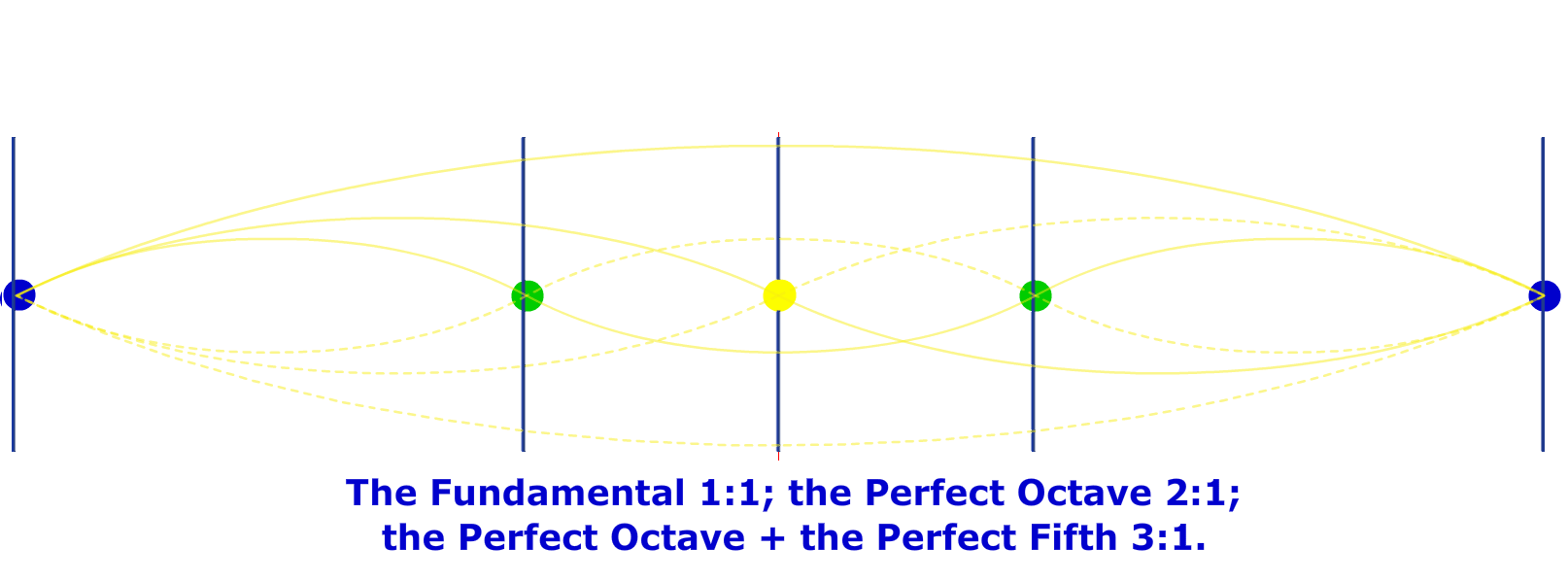Quantum wave:particle 'perceptions' of 'plureality',
a derived function of uncertainties,
often presented as probability-density-functions (pdfs) are cognitive-dissonance (noise).

Measurable per unit-time, Well Tempered Tuning (WTT) shows the derivation of 'Phi'

In music Well Tempered Tuning results from comparing . . .

7 Perfect Octaves & 12 Perfect 5ths (PF) which is
2^7=*128* : 1.5^12=129.7463379 where they don't commute
i.e. revealing the Pythagorean Comma (PC) = 1.7463379 . . . .

with

7 Perfect Octaves & 12 Well-Tempered 5ths (WTF) which is
2^7=*128* : 1.4983070768^12=*128* where they do commute
i.e. removing the Pythagorean Comma i.e. to = 0

This mirrors the Tao Te King of Lao-Tzi

Verse 42 of the Tao Te King reads (NB Hitchhiker's . . . )

"The Tao begot one. One begot two. Two begot three.
And three begot the ten thousand things.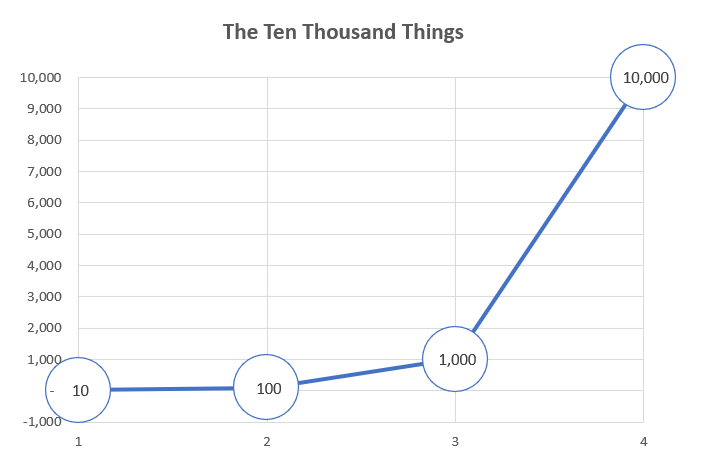The ten thousand things carry yin and embrace yang.
They achieve harmony by combining these forces."

Lao Tzi lived in China around 500 BCE. WTT is Pure Dao 84 verses long.
He was roughly contemporary with Pythagoras . . . so

1. the 84 verses of the Tao Te King equal 7 (Perfect Octaves) * 12 (Perfect Fifths) = 84 and
2. the 84 verses of the Tao Te King equal 7 (Perfect Octaves) * 12 (Well Tempered Fifths) = 84 and
3. feeding back the differences between 12 Perfect Fifths & 12 Well Tempered Fifths, leads to Phi at step 12.618

so . . .

1. the 'exponential growth curve' - Yang - acceleration from nought to infinity
2. creating the 'organic growth curve' - Yin - acceleration/deceleration between nought & 0.618 (at step 12.618)
3. when the differences-curve is fedback on the differences-curve, these show 'symmetry-binding' curvature
4. rescuing the Standard Model of particle physics, once gravity (the Higg's Boson/Field) is included
5. achieving harmony through the interplay of the forces of yin and yang, where any . . .
6. uncertainties are a function of the fundamental certainty from which they emerge.

The space-time based derivation of Phi, in 12 steps of the Pythagorean Comma from 0 to 0.618 is shown below.

It is . . .

1. not a probability distribution;
2. not a confidence interval;
3. not a measure of uncertainty.

QUANTRA

It is 'quantric' - over the 13.7 billion years since the Big Bang this fundamental cosmological consonance is . . .

4. 'quantric-certainty' with a high degree of accuracy which precedes and informs the
uncertainties of the Standard Model
5. and the cognitive dissonance in the uncertainties before & beyond atoms, molecules, evolution and the American Dream,
6. Phi-derivation
as demonstrated in the slide sequence and images above . . .

*************************************************************************

As for ‘avoiding extinction’ from Climate Change . . .

well it underlies C&C, The Well-Tempered Climate Accord.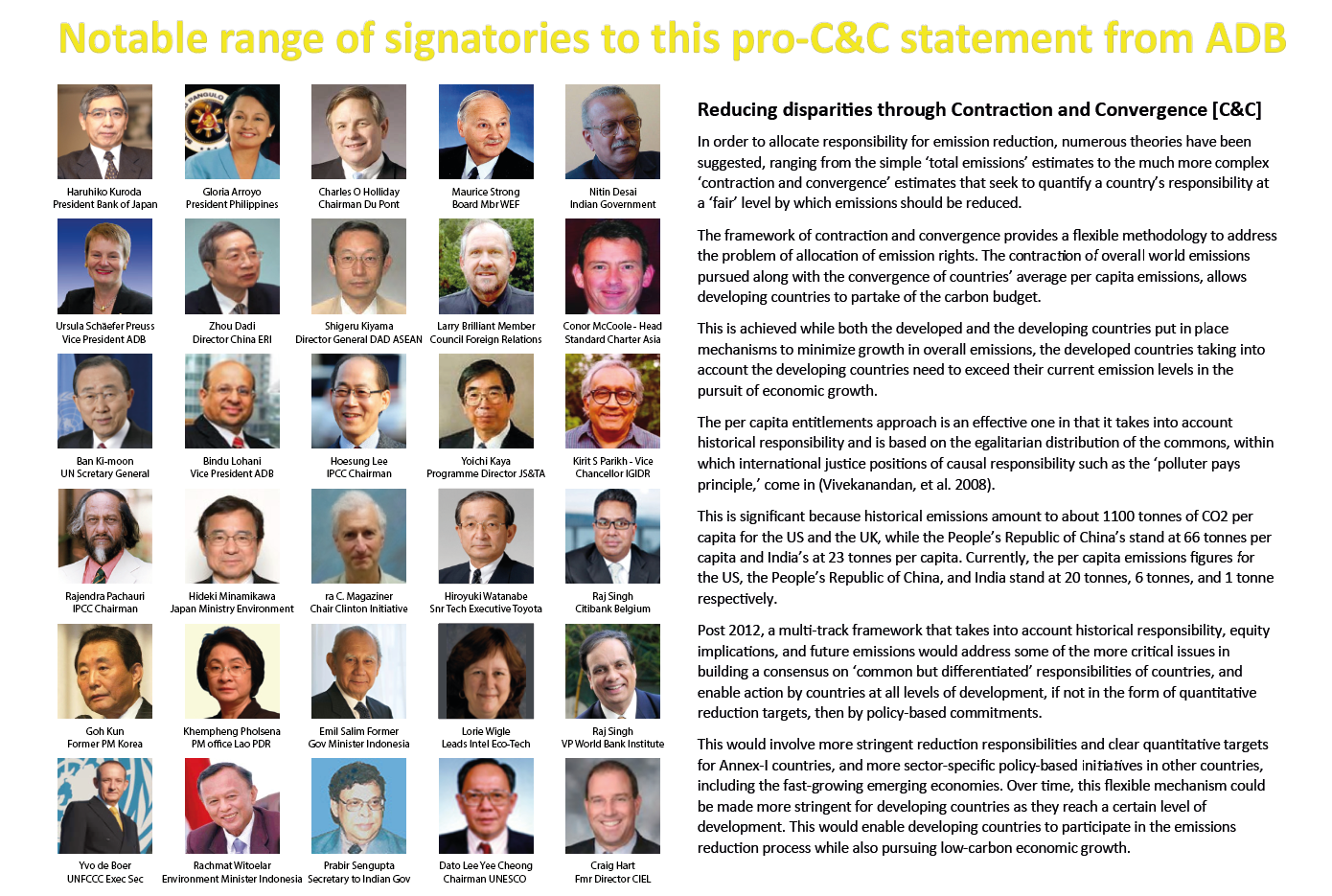There'n not much time left to achieve UNFCCC-Compliance now: -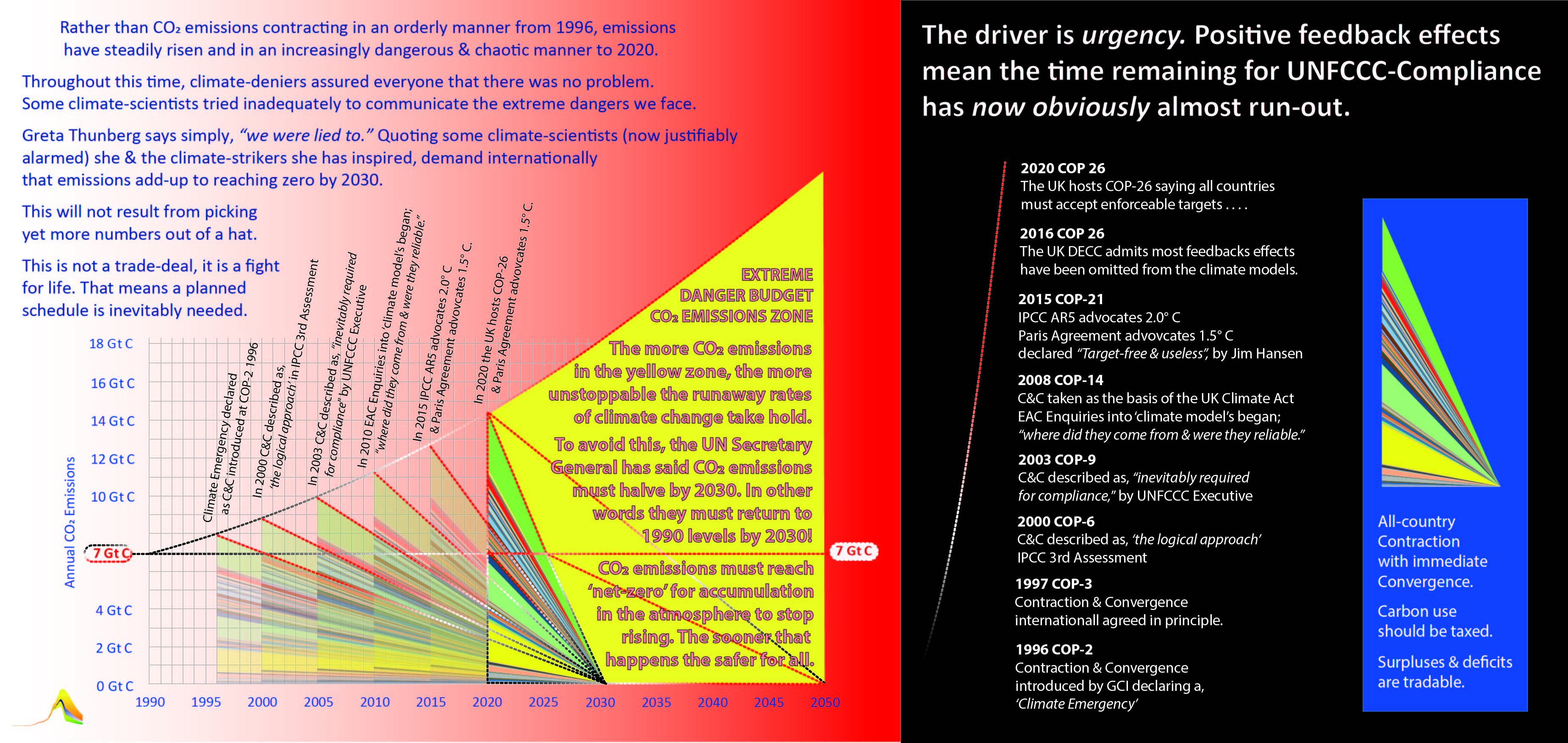What we need is an inclusive transparent full-term international strategy based on sharing the global commons"A superb example of how the arts put a great idea on the international stage."
John Vidal, Chairing an Ashden Theatres Trust Conference (+/- 2009)